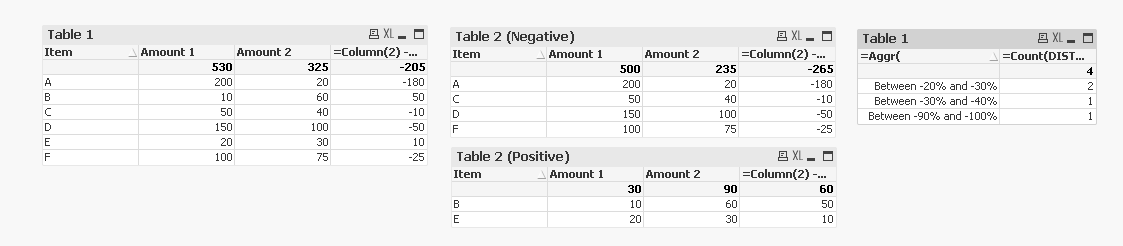# QlikView App Development

Discussion Board for collaboration related to QlikView App Development.

Contributor

## How to aggregate positive/negative values of expression

Let's say I load the following two tables :

ItemAmount 1Amount 2
A1000

B

0

40
C5040
D150100

ItemAmount 1Amount 2
A10020

B

10

20
E2030
F10075

I want to do the following :

1) Display a table with the difference D between Amount 2 and Amount1 (D = Amount 2 - Amount 1)

2) Display a table with only the lines where D >0, where D <0

3) Make and aggregation like : how many lines where D is negative and D/Amount 1 is between 0 and 10%, 10% and 20%, etc.

So

1) Display a table with the difference D betwenn Amount 2 and Amount1 (D = Amount 2 - Amount 1)

->it is easy, I use a table with Item as dimension, and 3 expressions (sum(Amount 1), sum(Amount 2), sum(Amount2)-Sum(Amount 1); this gives Something like

ItemAmount 1Amount 2D
A20020-180
B106050
C5040-10
D150100-50
E203010
F10075-25
TOTAL530325-205

2) Display a table with only the lines where D >0 or where D <0

How do I obtain this

ItemAmount 1Amount 2D
B106050
E203010
TOTAL309060

and this

ItemAmount 1Amount 2D
A20020-180
C5040-10
D150100-50
F10075-25
TOTAL500235-265

3) Make and aggregation like : nbr of lines where D is negative and D/Amount 1 is between 0 and 10%, 10% and 20%, etc.

How do I obtain this?

Decrease range # of items
Between 0% and -10%0
Between -10% and -20%0
Between -20% and -30%2 (*)
Between -30% and -40%1(**)
Between -40% and -50%0
Between -50% and -60%0
Between -60% and -70%0
Between -70% and -80%0
Between -80% and -90%0
Between -90% and -100%1 (***)

(*) is C and F

(**) id D

(***) is A

Olivier

3 RepliesMVP

## Re: How to aggregate positive/negative values of expression

Check the attachedContributor

## Re: How to aggregate positive/negative values of expression

Hi Sunny,

I am so impressed! Exactly what I was looking for...

Now if I may ask; could you explain to me a bit what is exactly going in the following lines :

=Sum({<Item = {"=Sum([Amount 2]) - Sum([Amount 1]) < 0"}>}[Amount 1])

it looks like a set analysis statement, but I don't get the "Item = Something being < 0"

Also I really don't get what's going on in the aggr dimension

Aggr(

If(Sum([Amount 2]) - Sum([Amount 1]) < 0,

If((Sum([Amount 2]) - Sum([Amount 1]))/Sum([Amount 1]) > -0.1, Dual('Between 0% and -10%', 1),

If((Sum([Amount 2]) - Sum([Amount 1]))/Sum([Amount 1]) > -0.2, Dual('Between -10% and -20%', 2),

If((Sum([Amount 2]) - Sum([Amount 1]))/Sum([Amount 1]) > -0.3, Dual('Between -20% and -30%', 3),

If((Sum([Amount 2]) - Sum([Amount 1]))/Sum([Amount 1]) > -0.4, Dual('Between -30% and -40%', 4),

If((Sum([Amount 2]) - Sum([Amount 1]))/Sum([Amount 1]) > -0.5, Dual('Between -40% and -50%', 5),

If((Sum([Amount 2]) - Sum([Amount 1]))/Sum([Amount 1]) > -0.6, Dual('Between -50% and -60%', 6),

If((Sum([Amount 2]) - Sum([Amount 1]))/Sum([Amount 1]) > -0.7, Dual('Between -60% and -70%', 7),

If((Sum([Amount 2]) - Sum([Amount 1]))/Sum([Amount 1]) > -0.8, Dual('Between -70% and -80%', 8),

If((Sum([Amount 2]) - Sum([Amount 1]))/Sum([Amount 1]) > -0.9, Dual('Between -80% and -90%', 9),

If((Sum([Amount 2]) - Sum([Amount 1]))/Sum([Amount 1]) > -1, Dual('Between -90% and -100%', 10)))))))))))), Item)

Thanks

OlivierMVP